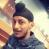true

Take Class 10 Tuition from the Best Tutors

•Affordable fees
•1-1 or Group class
•Flexible Timings
•Verified Tutors

Search in

# Introduction to current electricityRamneet Singh
19/04/2018 00

In our day to day life, we find the use of electricity for all our purposes. What is the concept behind the generation of current, where does it come from, is a matter of wonder to all us! Let us get introduced to how electricity is generated!

Current, as thought, conventionally flows in the direction of positive pole of electrode to negative electrode. By the later discoveries, with the help of technologies, it was found that the free electrons were the actual reason behind the flow of current. And surprisingly, it was found that the direction of eletrons flowing in a conductor was opposite to the direction of current flow.

Dear candidates who are appearing Class X, it's just an introduction, for full classes you may contact me!

Class IX students are also welcomed to join me at my classes.

Contact me on 9113436712.

0 Dislike
Follow 2## Other Lessons for You

Formula of A P
an = a+(n-1)d Where an=last term a=first term d=common difference n=number of term Note: last term is denoted by an or l Sn=n/2[2a+(n-1)d Sn=sum of all termsQ: The numerator of a fraction is 3 less than the denominator. If 2 is added to both the numerator and the denominator, then the sum of the new and the original fraction is 29/20. Find the...### Looking for Class 10 Tuition ?

Learn from Best Tutors on UrbanPro.

Are you a Tutor or Training Institute?

Join UrbanPro Today to find students near you

X

### Looking for Class 10 Tuition Classes?

The best tutors for Class 10 Tuition Classes are on UrbanPro

• Select the best Tutor
• Book & Attend a Free Demo
• Pay and start Learning### Take Class 10 Tuition with the Best Tutors

The best Tutors for Class 10 Tuition Classes are on UrbanPro